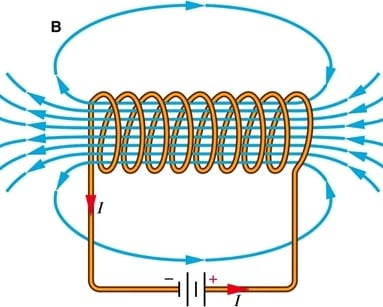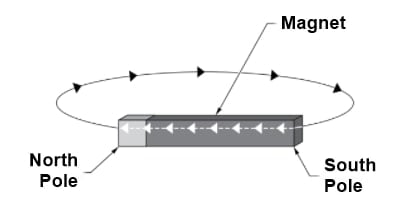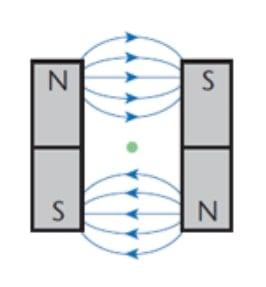Technical Article

# Understanding the Characteristics of Magnetic Lines of Force

September 06, 2021 by Alex Roderick

## This article will discuss how magnetic lines of force are related to currents in an electrical circuit.

Magnetic lines of force are created by current flowing in an electrical circuit. Unlike the current, magnetic lines of force are not considered as particles in motion but as a field of force exerted in space, similar to the force of gravity (see Figure 1).##### Figure 1. Magnetic lines of force help explain magnetism and the interaction of the electron with magnetic fields. Image courtesy of Brilliant

Despite the fact that magnetic lines of force are imaginary, they are important in describing magnetism and the interaction of electrons with magnetic fields. Magnetic lines of force are imaginary lines that represent the direction of the magnetic field as we move from one pole to the other.

The magnetic lines of force can be compared to rubber bands in that they contract when the magnetic force is removed and expand when the magnetic force is increased. Magnetic lines of force display the following characteristics:

• They are continuous loops.

• They cancel each other when traveling in opposite directions.

• They do not cross each other.

• They tend to take the path of least reluctance.

Magnetic lines of force form a complete loop and are continuous (see Figure 2). This implies that a single magnetic line of force leaves the north pole of a magnet, travels to the south pole, and completes the loop back to the north pole via the magnetic material. This is similar to current flow in an electrical circuit where electrons leaving the negative terminal of an electrical source return to the positive terminal of the source. Within the electrical source, the electrons move from the positive pole to the negative pole. Neither the magnetic lines of force nor the electrons disappear.##### Figure 2. Magnetic lines of force form a complete loop and are continuous.

The opposite poles of magnets attract each other whereas like poles repel one another. The magnetic lines of force are denser at the poles of a magnet.

Parallel magnetic lines of force that travel in opposite directions cancel each other. This is best explained using two current-carrying parallel conductors. The magnetic fields caused by electron flow run in opposite directions between the conductors. When the conductors are brought close enough for their fields to interact, the magnetic lines of force between the conductors cancel each other out. The magnetic lines of force that surround both conductors pull them together. Figure 4 below shows the field pattern between two magnets. As you can see, the magnetic lines of force traveling in opposite directions cancel each other at the midpoint and create a neutral point where the overall magnetic field is equal to zero.  Also, note that the magnetic lines of force do not cross each other.##### Figure 4. Lines traveling in opposite directions cancel one another. Image courtesy of Revision Science

Note

The fact that magnets always orient themselves in the same direction when free to turn shows that the Earth must also be a giant magnet. Otherwise, the same pole of a magnet would not always align in the same direction.

Magnetic lines of force tend to follow the path of least reluctance. Reluctance (R) is a concept used in the analysis of magnetic circuits. It is to specify how a given magnetic path opposes magnetic lines of force. Reluctance is directly proportional to the length and inversely proportional to the permeability and cross-sectional area of the material through which the flux travels. The unit of reluctance, which has no given name, is expressed by the following equation:

$\Re =\frac{l}{A\times \mu}$

where

$$\Re$$ = reluctance

$$l$$ = length (in meters)

$$A$$ = cross-sectional area (in square meters)

$$μ$$ = permeability

We can say that magnetic lines of force have elasticity. The pattern of magnetic field lines changes when a conductor passes through them and returns to their original shape when the conductor has passed.

Magnetic lines of force can be harmful to some equipment. One method of shielding is to take advantage of the fact that magnetic lines of force tend to take the path of least reluctance. This principle is often used to protect watch movements. The watch movement is protected from becoming magnetized by being enclosed in a low reluctance material that allows the magnetic field to bypass the watch movement.

Faraday developed a visual model of the magnetic field. He theorized that moving a magnet or an electrical charge disturbs the magnetic lines of force surrounding the magnet or charge. He concluded that this effect travels at a definite speed. In 1864, James Clerk Maxwell, a Scottish physicist, published his mathematical theory that the changes in these fields could be treated as a wave motion and travel at the speed of light (300 × 106 m/s). Maxwell also came to the conclusion that light is an electromagnetic wave and all such waves consist of the growth and collapse of electric and magnetic fields at right angles to each other. The concentric magnetic lines of force can travel in the clockwise or counterclockwise direction depending on the direction of electron flow through a conductor.